﻿ 基于投影寻踪动态聚类模型的城市内涝风险评估 Urban Waterlogging Risk Assessment Based on Projection Pursuit Dynamic Clustering Model

Computer Science and Application
Vol. 09  No. 04 ( 2019 ), Article ID: 29623 , 8 pages
10.12677/CSA.2019.94076

Urban Waterlogging Risk Assessment Based on Projection Pursuit Dynamic Clustering Model

Jiangtao Wang

Shanghai Maritime University, ShanghaiReceived: Mar. 20th, 2019; accepted: Apr. 2nd, 2019; published: Apr. 9th, 2019ABSTRACT

Urban flood risk assessment is critical to the prediction and reduction of flood disaster, and also the mitigation of drainage facilities. However, for flood risk assessment using multiple indicators, the index weight has a great impact on the results of flood risk assessment. In this paper, projection pursuit dynamic cluster method is used in order to reduce the uncertainties of the index weight. Projection pursuit dynamic cluster is a relatively objective method, which can evaluate the sample data comprehensively, and can also consider the preference of decision makers by setting constraint conditions. The SWMM model was established for a drainage system. Then different values for the evaluation index were got through simulation. Then the results of urban waterlogging risk assessment are obtained by the dynamic iteration between clustering center and relative membership degree. Through the analysis of the results, it shows that projection pursuit dynamic cluster method can be used for urban waterlogging risk assessment. Furthermore, it brings important guiding significance for urban disaster prevention and mitigation.

Keywords:Drainage System, Projection Pursuit, Dynamic Cluster, Variable Fuzzy Clustering, Risk Assessment1. 引言

21世纪，城市综合防灾减灾能力大小将成为衡量城市整体功能及其安全防卫能力的标志  。城市内涝灾害是由极端暴雨在短时间内超过雨水系统设计标准且低于城市防洪标准所导致的，具有持续时间短等特点。因此，对城市内涝形成机理和风险评估开展研究，为城市洪涝灾情预判工作提供及时有效的数据及信息，根据不同重现期的内涝灾害制定应急预案，对提高城市防灾减灾能力具有重要意义。

2. 投影寻踪动态聚类原理

1) 对各指数据进行无量纲化处理，即归一化，如公式(1)~(2)所示。

${x}_{ij}=\frac{{x}_{ij}^{0}-{x}_{j\mathrm{min}}^{0}}{{x}_{j\mathrm{max}}^{0}-{x}_{j\mathrm{min}}^{0}}$ (1)

${x}_{ij}=\frac{{x}_{j\mathrm{max}}^{0}-{x}_{ij}^{0}}{{x}_{j\mathrm{max}}^{0}-{x}_{j\mathrm{min}}^{0}}$ (2)

2) 投影可以从不同角度观察数据，寻找能够最大程度地反映高维数据特征和最能充分挖掘数据信息的最佳观察角度即最优投影方向。本文采用线性投影，即将高维数据投影到线性空间。设a为m维单位投影方向向量，其分量为 ${a}_{1},{a}_{2},\cdots ,{a}_{m}$，则xij的投影特征值zi可表示为

${z}_{i}=\sum {a}_{j}{x}_{ij}\text{\hspace{0.17em}}\text{\hspace{0.17em}}\left(i=1,\cdots ,n\right)$ (3)

3) 本文应用动态聚类方法来构造投影寻踪动态聚类模型的投影指标。假设整个样本的投影特征值序列组成的集合为 $\Omega =\left\{{z}_{1},{z}_{2},\cdots ,{z}_{n}\right\}$，依据实际情况或要求，采用动态聚类法将其聚为p (p ≤ n)类  ，具体实现步骤如下：

①随机选取p个点作为p个聚核，记为

${L}^{0}=\left({A}_{1}^{0},{A}_{2}^{0},\cdots ,{A}_{p}^{0}\right)$ (4)

②根据L0，把Ω中的点分为p类，记为

${\Theta }^{0}=\left({\Theta }_{1}^{0},{\Theta }_{2}^{0},\cdots ,{\Theta }_{p}^{0}\right)$ (5)

③从L0出发，得到新的聚核L1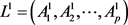(6)

④重复以上步骤，由此得到一个分类结果序列 ${V}^{k}=\left({L}^{k},{\Theta }^{k}\right),k=1,2,\cdots$，记

$D\left({A}_{i}^{k},{\Theta }_{i}^{k}\right)=\underset{{z}_{i}\in {\Theta }_{i}^{k}}{\sum }|{z}_{i}-{A}_{i}^{k}|$ (7)

${u}_{k}=\underset{i=1}{\overset{p}{\sum }}D\left({A}_{i}^{k},{\Theta }_{i}^{k}\right)$ (8)

$\frac{|{u}_{k+1}-{u}_{k}|}{{u}_{k+1}}\le \epsilon$ 时终止计算，其中ɛ是一个充分小的误差允许值。

${\Theta }_{h}\left(h=1,2,\cdots ,p\right)$ 为由属于第h类的所有样本投影特征值构成的集合，定义 $d\left({z}_{i},{z}_{j}\right)$ 为任意两投影特征值间的绝对值距离。为了表示类内样本的邻近程度，则用如下公式表示

$dd\left(a\right)=\underset{h=1}{\overset{p}{\sum }}{D}_{h}\left(a\right)$ (9)

${D}_{h}\left(a\right)=\underset{{z}_{i},{z}_{j}\in {\Theta }_{h}}{\sum }d\left({z}_{i},{z}_{j}\right)$ (10)

$ss\left(a\right)=\underset{{z}_{i},{z}_{j}\in Q}{\sum }d\left({z}_{i},{z}_{j}\right)$ (11)

$QQ\left(a\right)=ss\left(a\right)-dd\left(a\right)$ (12)

4) 模型求解。

$\left\{\begin{array}{l}\mathrm{max}QQ\left(a\right)\\ ‖a‖=1\end{array}$ (13)

3. 实例分析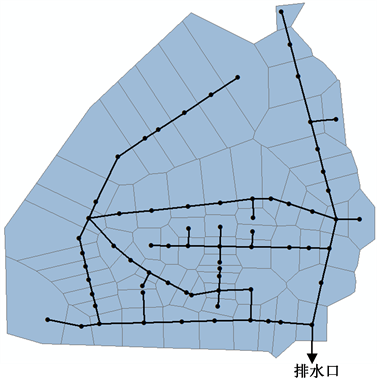Figure 1. Drainage system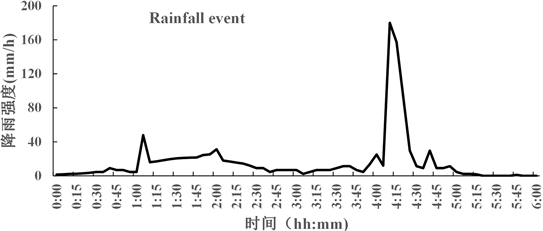Figure 2. 6 h rainfall hyetographs of the 6 August 2014 event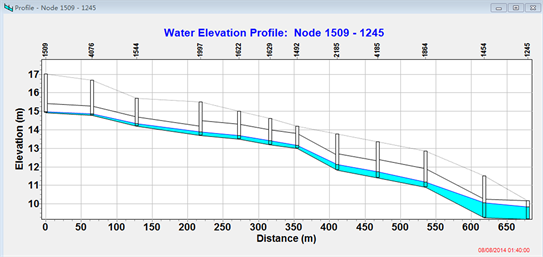Figure 3. Water level diagram of some pipes at 01:40:00Table 1. Flood risk evaluation factor sample set and values of projection and risk degree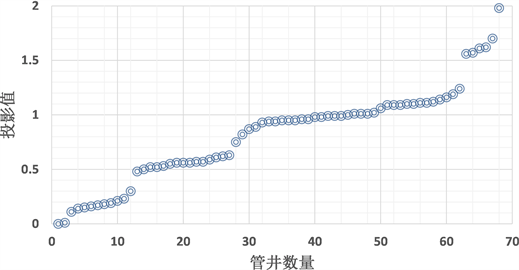Figure 4. Projection values of different wells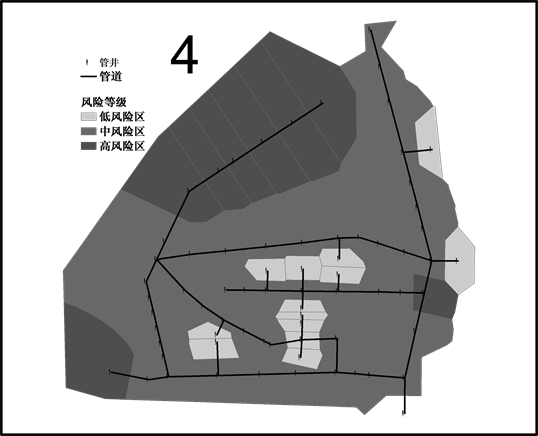Figure 5. Flood risk map of the study area

4. 结语

Urban Waterlogging Risk Assessment Based on Projection Pursuit Dynamic Clustering Model[J]. 计算机科学与应用, 2019, 09(04): 674-681. https://doi.org/10.12677/CSA.2019.94076

1. 1. 周振民. 城市水务学[M]. 北京: 科学出版社, 2013.

2. 2. 谢映霞. 从城市内涝灾害频发看排水规划的发展趋势[J]. 城市规划, 2013(2): 45-50.

3. 3. 金磊. 城市灾害学原理[M]. 北京: 气象出版社, 1997.

4. 4. 孙章丽, 朱秀芳, 潘耀忠, 等. 洪水灾害风险分析进展与展望[J]. 灾害学, 2017(3): 125-130+136.

5. 5. 黄维. 城市排水管网水力模拟及内涝风险评估[D]: [硕士学位论文]. 广州: 华南理工大学, 2016.

6. 6. 戴晶晶, 刘增贤, 陆沈钧. 基于数值模拟的城市内涝风险评估研究——以苏州市城市中心区为例[J]. 中国水利, 2015(13): 20-23.

7. 7. 黄铁兰, 陈小芬, 苏章灿. 基于遥感和GIS的城市内涝风险评价——以广州市天河区为例[J]. 人民珠江, 2017(2): 89-95.

8. 8. 王磊, 周玉文. 基于投影寻踪的城市排水系统洪涝风险评估模型[J]. 中国给水排水, 2011, 27(23): 78-82.

9. 9. Friedman, J.H. and Tukey, J.W. (1974) A Projection Pursuit Algorithm for Exploratory Data Analysis. IEEE Transactions on Computers, 23, 881-890. https://doi.org/10.1109/T-C.1974.224051

10. 10. Glover, D.M. and Hopke, P.K. (1994) Exploration of Multivariate Atmospheric Particulate Compositional Data by Projection Pursuit. Atmospheric Environment, 28, 1411-1424. https://doi.org/10.1016/1352-2310(94)90204-6

11. 11. 倪长健, 王顺久, 崔鹏. 投影寻踪动态聚类模型及其在地下水分类中的应用[J]. 四川大学学报(工程科学版), 2006, 38(6): 29-33.

12. 12. 王卓, 倪长健. 投影寻踪动态聚类模型研究及其在洪灾评定中的应用[J]. 四川师范大学学报(自然科学版), 2008, 31(5): 635-638.

13. 13. 任若恩, 王惠文. 多元统计数据分析: 理论、方法、实例[M]. 北京: 国防工业出版社, 1997.

14. 14. 丛翔宇, 倪广恒, 惠士博, 等. 基于SWMM的北京市典型城区暴雨洪水模拟分析[J]. 水利水电技术, 2006(4): 64-67.

15. 15. 符锐, 罗龙洪, 刘俊, 等. SWMM模型中的低影响开发模块在排水防涝系统中的应用[J]. 水电能源科学, 2014(9): 71-74.

16. 16. 王慧亮, 吴泽宁, 胡彩虹, 等. 基于GIS与SWMM耦合的城市暴雨洪水淹没分析[J]. 人民黄河, 2017(8): 31-35+43.